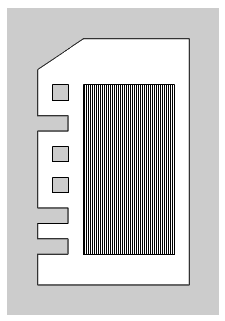# Microfiches

Time Limit: Java: 2000 ms / Others: 2000 ms

Memory Limit: Java: 65536 KB / Others: 65536 KB

## Description

Before computers were widely used, there was another fairly convenient way of archiving data, where librarians archived periodicals on microfiches. A microfiche is a rectangular card made of high-resolution magnet that can store the image of a newspaper page on an area as small as one square millimeter. One may view the newspaper from a microfiche by a device with strong magnification capability. In order to keep a large microfiche collection in perfect order and provide good search service to the users, Gholam, one ingenious librarian, invented a microfiche storage machine.

There are n microfiches in the collection, and each of them has a unique id number. There is a catalogue that allows one to easily find the id number of the microfiche desired.

Gholam proposes to put each microfiche in a special frame, as shown in the figure below. The upper left corner of the frame is clipped, to make sure that there is only one orientation possible for a framed microfiche in its stack (explained below).Figure 1: A microfiche with id number 010011, mounted on a frame. The shaded black portion is the microfiche and the surrounding white part is the frame containing the microfiche.

The id number of a microfiche is encoded, in binary, on the frame as follows. Let g be the number of bits necessary to represent an id number, indexed from 0 to g - 1. The left side of the frame contains g information cells. A "1" is represented as a dent on the information cell, and a "0" is represented as a hole. The first (uppermost) information cell corresponds to the most significant bit of the id number; the last information cell corresponds to its least significant bit. See the above figure for an illustration.

The microfiche storage machine contains three stacks, stack 0, stack 1, and stack 2, capable of holding n microfiches each. If you look at these stacks from the top, each of them is shaped like a rectangle with the upper left corner clipped. Initially, stack 0 is used for storage of microfiches; stacks 1 and 2 are only used for performing the move operation described below. The machine used to perform more operations, but move is the only operation that is considered in this problem.Figure 2: The microfiche with a hole in position 3 gets pulled at (A), and the one with a dent in position 3 stays in the stack (B)

move (i, k, j): This operation moves all the microfiches with a "0" in information cell k (0 k g - 1) from stack i to the top of stack j such that the original relative ordering of the pulled microfiches from stack i , and that of the ones originally in stack j are preserved. In practice, a rod is inserted through all the holes (encoded "0"s) and dents (encoded "1"s) of the information cells in position k. Then the machine pulls the rod to the left, and thus the microfiches that have a hole (a "0") at position k will be pulled out (e.g. the microfiche of Figure 2.A), but those which have a dent ("1") in position k (e.g. Fig 2.B) would be left in the stack.

Gholam wants to find a certain microfiche with a known id number. He achieves this goal by performing a sequence of move operations, such that after performing the operations, there will be one stack that holds the desired microfiche only. Your task is to write a program to find the minimum length of such a sequence.

## Input

The first line of the input file contains a single integer t (1 <= t <= 10), the number of test cases, followed by the input data for each test case. The first line of each test data are two integers n (1 <= n <= 10000) and g (1 <= g <= 15) which are the number of microfiches and the number of bits, respectively. Following the first line, there are n lines each containing the binary representation of a g-bit integer, with no leading or trailing spaces. The desired microfiche is the first one appearing in the test case.

## Output

There should be one output line per test case containing the minimum number of move operations needed to find the desired microfiche.

## Sample Input

1
4 3
010
011
100
110

## Sample Output

2

None

## Source

Asia 2002, Tehran (Iran), Preliminary